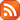Notice: Important information about COVID-19 here.Subscribe by RSS

# Seminar: Mathematical Aspects of Quantum Measurement

Fri., Mar. 8, 2013 3:30 p.m. - Fri., Mar. 8, 2013 4:30 p.m.

Location: RIC 208

The probabilistic aspects of quantum theory have led mathematical and theoretical physicists to consider so-called quantum analogues of commonly used notions in classical probability theory. By the term "quantum&" one usually means "operator" valued. The theory of positive operator valued measures appears explicitly in quantum theory via the measurement postulate, which is meant to capture the fact that obtaining information about a quantum system in a given state generally alters the state of the system. This quantisation of classical probability theory leads to a number of new mathematical structures, some of which I will describe in this lecture and are of interest in quantum information theory.

Speaker: Dr. Doug Farenick, Department of Mathematics and Statistics, University of Regina

Please download our poster (440 kB)format.